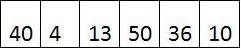# Selection Sort, the Basic Sorting Algorithm

Written by Raza on. Posted in C++

Selection sort is one of the basic sorting Algorithms. It is pretty simple to understand and very good to implement on small amount of data. However, it becomes quite inefficient when working with large lists.

Selection Sort uses the concept of in-place sorting. It means that the data is sorted by shuffling it within the list. First I shall try to explain the algorithm and then together we would try to carve out of it a basic source code.

## Algorithm :

It consists of two major parts.

1. Finding the minimum or the maximum value in the given list.
2. Swapping it with the value at the first or the last index.

We would repeat this procedure for the complete List and in each cycle, we would decrease the size of the List we are working on, from the left hand side. Why? Because when we have got the smallest value located in its proper place, then we try to bring other values to their correct places as well.

Notice that if we are trying to sort in ascending order (1.2.3.4.5.6), we would find the minimum value and place it at the beginning of the list. If we are sorting in descending order (6.5.4.3.2.1), we would find the minimum value and place it at the end of the list.

Anyways, we shall sort in ascending order considering our List to be an Array of integers.

Consider we have this list.So, to sum it up, what we have done is that we online casino took an Array, found out the smallest value in it and placed it at the first position. Then we found the second highest value and placed it at the second position, and so on until we sorted out the whole Array.

One limitation of this Algorithm comes into account when the Array in hand is already sorted. In this case, we still have to perform all the procedure as in the normal case. We don’t have any method which tells us that whether the array sorted or not? In some other Algorithms, however, we could find it out in the first step which saves us a lot of time and energy.

## Source Code :

With the Analysis of the Algorithm done, we try to figure out a basic source code on its basis.

```</p>
<p>const int SIZE = 10 ; &nbsp; &nbsp; &nbsp; //Size of the Array</p>
<p>int arr [SIZE] = {-1,9,4,-10,6,5,8,-3,2,7} ; &nbsp; &nbsp; &nbsp; &nbsp; &nbsp;//Array of SIZE 10</p>
<p>int min ; &nbsp; &nbsp; &nbsp; &nbsp; &nbsp; &nbsp; &nbsp;//Minimum value in each step</p>
<p>for (int i = 0 ; i &lt; SIZE - 1 ; i) &nbsp; &nbsp; &nbsp; &nbsp; &nbsp; &nbsp; //Loop which runs over the Array</p>
<p>{</p>
<p>&nbsp; &nbsp; &nbsp; &nbsp; min = i ; &nbsp; &nbsp; &nbsp; &nbsp; &nbsp; &nbsp; //Suppose it to be equal to value at index i</p>
<p>&nbsp; &nbsp; &nbsp; &nbsp; for (int j = i 1 ; j &lt; SIZE ; j) &nbsp; &nbsp; &nbsp;//Loop which finds the minimum value &nbsp; &nbsp; &nbsp; &nbsp; &nbsp; &nbsp;</p>
<p>&nbsp; &nbsp; &nbsp; &nbsp; &nbsp; { &nbsp; &nbsp; &nbsp; &nbsp; &nbsp; &nbsp; &nbsp;</p>
<p>&nbsp; &nbsp; &nbsp; &nbsp; &nbsp; &nbsp; &nbsp; &nbsp;if (arr [min] &gt; arr [j]) &nbsp; &nbsp; //If supposed value is not smaller</p>
<p>&nbsp; &nbsp; &nbsp; &nbsp; &nbsp; &nbsp; &nbsp; &nbsp; {</p>
<p>&nbsp; &nbsp; &nbsp; &nbsp; &nbsp; &nbsp; &nbsp; &nbsp; &nbsp; &nbsp; min = j ;</p>
<p>&nbsp; &nbsp; &nbsp; &nbsp; &nbsp; &nbsp; &nbsp; &nbsp; }</p>
<p>&nbsp; &nbsp; &nbsp; &nbsp; &nbsp;}</p>
<p>&nbsp; &nbsp; &nbsp; &nbsp; &nbsp;if (min != i) &nbsp; &nbsp; &nbsp; //if minimum number is not equal the the supposed number</p>
<p>&nbsp; &nbsp; &nbsp; &nbsp; &nbsp;{</p>
<p>&nbsp; &nbsp; &nbsp; &nbsp; &nbsp; &nbsp; &nbsp; &nbsp;swap (arr [i], arr [min]) ; &nbsp; &nbsp; &nbsp; &nbsp; &nbsp; &nbsp; &nbsp; &nbsp;//swaps their values</p>
<p>&nbsp; &nbsp; &nbsp; &nbsp; &nbsp;}</p>
<p>}</p>
<p>```

There could be many ways of Writing the source code. This one is simple to understand. You can write it in your own way which could be even better than this one. Feel free to post it here! Next time I would be sharing this very source code written in a recursive manner. Are you familiar with recursion? No. Yes. Even if yes, I would be exploring the recursive phenomena and then deal with selection sort via recursion.

Switch to Desktop Version